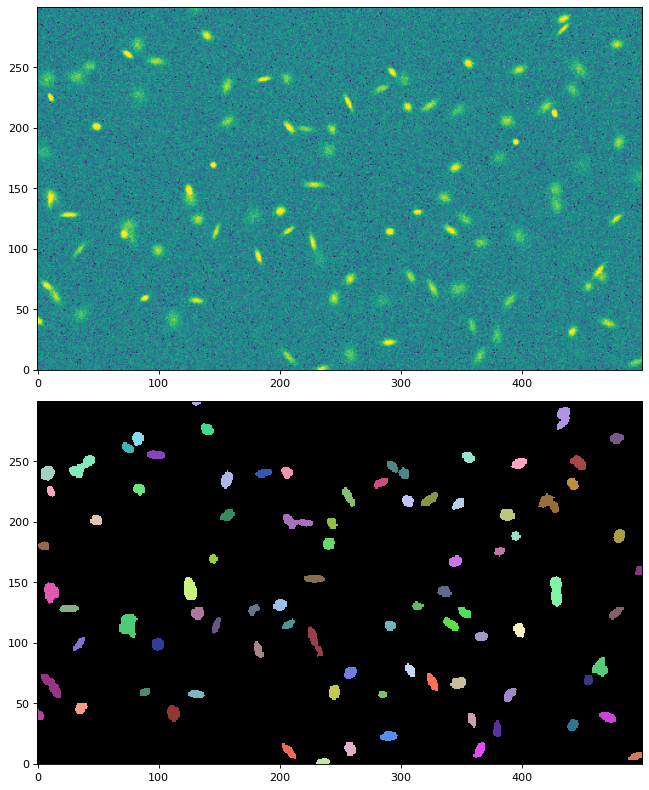# detect_sources¶

`photutils.segmentation.``detect_sources`(data, threshold, npixels, filter_kernel=None, connectivity=8, mask=None)[source]

Detect sources above a specified threshold value in an image and return a `SegmentationImage` object.

Detected sources must have `npixels` connected pixels that are each greater than the `threshold` value. If the filtering option is used, then the `threshold` is applied to the filtered image. The input `mask` can be used to mask pixels in the input data. Masked pixels will not be included in any source.

This function does not deblend overlapping sources. First use this function to detect sources followed by `deblend_sources()` to deblend sources.

Parameters
dataarray_like

The 2D array of the image.

thresholdfloat or array-like

The data value or pixel-wise data values to be used for the detection threshold. A 2D `threshold` must have the same shape as `data`. See `detect_threshold` for one way to create a `threshold` image.

npixelsint

The number of connected pixels, each greater than `threshold`, that an object must have to be detected. `npixels` must be a positive integer.

filter_kernelarray-like (2D) or `Kernel2D`, optional

The 2D array of the kernel used to filter the image before thresholding. Filtering the image will smooth the noise and maximize detectability of objects with a shape similar to the kernel.

connectivity{4, 8}, optional

The type of pixel connectivity used in determining how pixels are grouped into a detected source. The options are 4 or 8 (default). 4-connected pixels touch along their edges. 8-connected pixels touch along their edges or corners. For reference, SourceExtractor uses 8-connected pixels.

maskarray_like of bool, optional

A boolean mask, with the same shape as the input `data`, where `True` values indicate masked pixels. Masked pixels will not be included in any source.

Returns
segment_image

A 2D segmentation image, with the same shape as `data`, where sources are marked by different positive integer values. A value of zero is reserved for the background. If no sources are found then `None` is returned.

Examples

```# make a table of Gaussian sources
from astropy.table import Table
table = Table()
table['amplitude'] = [50, 70, 150, 210]
table['x_mean'] = [160, 25, 150, 90]
table['y_mean'] = [70, 40, 25, 60]
table['x_stddev'] = [15.2, 5.1, 3., 8.1]
table['y_stddev'] = [2.6, 2.5, 3., 4.7]
table['theta'] = np.array([145., 20., 0., 60.]) * np.pi / 180.

# make an image of the sources with Gaussian noise
from photutils.datasets import make_gaussian_sources_image
from photutils.datasets import make_noise_image
shape = (100, 200)
sources = make_gaussian_sources_image(shape, table)
noise = make_noise_image(shape, distribution='gaussian', mean=0.,
stddev=5., seed=0)
image = sources + noise

# detect the sources
from photutils.segmentation import detect_threshold, detect_sources
threshold = detect_threshold(image, nsigma=3)
from astropy.convolution import Gaussian2DKernel
kernel_sigma = 3.0 / (2.0 * np.sqrt(2.0 * np.log(2.0)))  # FWHM = 3
kernel = Gaussian2DKernel(kernel_sigma, x_size=3, y_size=3)
kernel.normalize()
segm = detect_sources(image, threshold, npixels=5,
filter_kernel=kernel)

# plot the image and the segmentation image
import matplotlib.pyplot as plt
fig, (ax1, ax2) = plt.subplots(2, 1, figsize=(8, 8))
ax1.imshow(image, origin='lower', interpolation='nearest')
ax2.imshow(segm.data, origin='lower', interpolation='nearest')
```